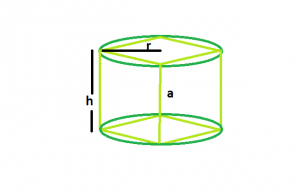# Largest cube that can be inscribed within a right circular cylinder

Given here is a right circular cylinder of height h and radius r. The task is to find the volume of biggest cube that can be inscribed within it.

Examples:

```Input: h = 3, r = 2
Output: volume = 27

Input: h = 5, r = 4
Output: volume = 125
```## Recommended: Please try your approach on {IDE} first, before moving on to the solution.

Approach: From the figure, it can be clearly understand that side of the cube = height of the cylinder.
So, the volume = (height)^3

Below is the implementation of the above approach:

## C++

 `// C++ Program to find the biggest cube ` `// inscribed within a right circular cylinder ` `#include ` `using` `namespace` `std; ` ` `  `// Function to find the volume of the cube ` `float` `cube(``float` `h, ``float` `r) ` `{ ` ` `  `    ``// height and radius cannot be negative ` `    ``if` `(h < 0 && r < 0) ` `        ``return` `-1; ` ` `  `    ``// volume of the cube ` `    ``float` `a = ``pow``(h, 3); ` ` `  `    ``return` `a; ` `} ` ` `  `// Driver code ` `int` `main() ` `{ ` `    ``float` `h = 5, r = 4; ` `    ``cout << cube(h, r) << endl; ` ` `  `    ``return` `0; ` `} `

## Java

 `// Java Program to find the biggest cube  ` `// inscribed within a right circular cylinder  ` `class` `Solution ` `{ ` `     `  ` `  `// Function to find the volume of the cube  ` `static` `float` `cube(``float` `h, ``float` `r)  ` `{  ` ` `  `    ``// height and radius cannot be negative  ` `    ``if` `(h < ``0` `&& r < ``0``)  ` `        ``return` `-``1``;  ` ` `  `    ``// volume of the cube  ` `    ``float` `a = (``float``)Math.pow(h, ``3``);  ` ` `  `    ``return` `a;  ` `}  ` ` `  `// Driver code  ` `public` `static` `void` `main(String args[]) ` `{  ` `    ``float` `h = ``5``, r = ``4``;  ` `    ``System.out.println( cube(h, r) );  ` `} ` `}  ` `//contributed by Arnab Kundu `

## Python 3

 `# Python 3 Program to find the biggest cube ` `# inscribed within a right circular cylinder ` `import` `math ` ` `  `# Function to find the volume of the cube ` `def` `cube(h, r): ` ` `  `    ``# height and radius cannot be negative ` `    ``if` `(h < ``0` `and` `r < ``0``): ` `        ``return` `-``1` ` `  `    ``# volume of the cube ` `    ``a ``=` `math.``pow``(h, ``3``) ` ` `  `    ``return` `a ` ` `  `# Driver code ` `h ``=` `5``; r ``=` `4``; ` `print``(cube(h, r)); ` ` `  `# This code is contributed ` `# by Akanksha Rai `

## C#

 `// C# Program to find the biggest ` `// cube inscribed within a right  ` `// circular cylinder  ` `using` `System; ` `                     `  `class` `GFG ` `{ ` ` `  `// Function to find the volume ` `// of the cube  ` `static` `float` `cube(``float` `h, ``float` `r)  ` `{  ` ` `  `    ``// height and radius cannot ` `    ``// be negative  ` `    ``if` `(h < 0 && r < 0)  ` `        ``return` `-1;  ` ` `  `    ``// volume of the cube  ` `    ``float` `a = (``float``)Math.Pow(h, 3);  ` ` `  `    ``return` `a;  ` `}  ` ` `  `// Driver code  ` `public` `static` `void` `Main() ` `{  ` `    ``float` `h = 5, r = 4;  ` `    ``Console.Write( cube(h, r) );  ` `} ` `} ` ` `  `// This code is contributed  ` `// by 29AjayKumar `

## PHP

 ` `

Output:

```125
```

Don’t stop now and take your learning to the next level. Learn all the important concepts of Data Structures and Algorithms with the help of the most trusted course: DSA Self Paced. Become industry ready at a student-friendly price.

My Personal Notes arrow_drop_upCheck out this Author's contributed articles.

If you like GeeksforGeeks and would like to contribute, you can also write an article using contribute.geeksforgeeks.org or mail your article to contribute@geeksforgeeks.org. See your article appearing on the GeeksforGeeks main page and help other Geeks.

Please Improve this article if you find anything incorrect by clicking on the "Improve Article" button below.

Article Tags :
Practice Tags :

Be the First to upvote.

Please write to us at contribute@geeksforgeeks.org to report any issue with the above content.